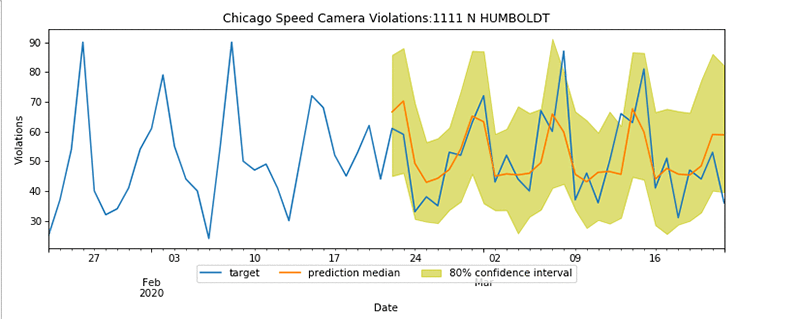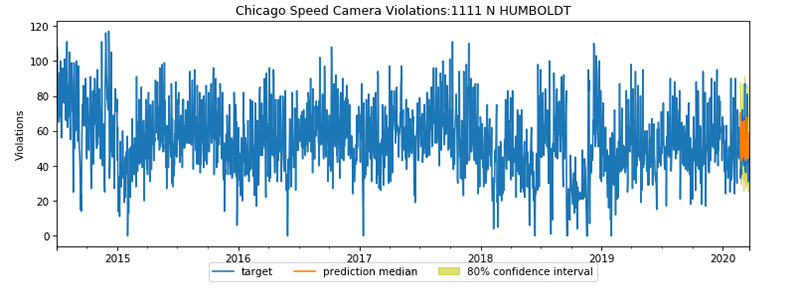### Echo Dot (3rd Gen) - Smart speaker with Alexa - Charcoal

Use your voice to play a song, artist, or genre through Amazon Music, Apple Music, Spotify, Pandora, and others. With compatible Echo devices in different rooms, you can fill your whole home with music.### Wireless Rechargeable Battery Powered WiFi Camera.

Wireless Rechargeable Battery Powered WiFi Camera is home security camera system lets you listen in and talk back through the built in speaker and microphone that work directly through your iPhone or Android Mic.

# Predicting driving speed violations with the Amazon SageMaker DeepAR algorithm

0
371Forecasting is an important aspect for many businesses and industries; forging ahead without clearly defined goals could have serious consequences. Product planning, financial forecasting, and weather forecasting create scientific estimates based on hard data and critical analysis. Time-series forecasting decomposes the historical data into the baseline, trend, and seasonality, if any.

The Amazon SageMaker DeepAR forecasting algorithm is a supervised machine learning algorithm to forecast a time series. The algorithm uses recurrent neural networks (RNN) to produce point and probabilistic forecasts. You can use the DeepAR algorithm for forecasting single values for a scalar (one-dimensional) time series, or have it work on hundreds of related time series simultaneously by creating a model. It can even predict a new time series that is related to the series on which the model is trained.

To illustrate time series forecasting, I use the DeepAR algorithm to analyze Chicago’s Speed Camera Violation dataset. The dataset is hosted by Data.gov and managed by the U.S. General Services Administration, Technology Transformation Service. These violations are captured by camera systems and available on the Chicago Data Portal. You can use the dataset to discern patterns in the data and gain meaningful insights.

The dataset contains multiple camera locations and daily violation counts. If you imagine each daily violation for a camera as one time series, you can use the DeepAR algorithm to train a model for multiple streets simultaneously and predict violations for multiple street cameras.

This analysis can identify streets where motorists are most likely to drive above the speed limit at different times of year, and any seasonality in the data. This could help cities to implement proactive measures to reduce speed, create alternate routes, and increase safety.

The code for this notebook is available on the GitHub repo.

## Creating a Jupyter notebook

Before you get started, create an Amazon SageMaker Jupyter notebook instance. For this post, I use an ml.m4.xlarge notebook instance and built-in python3 kernel.

Download the data to the Jupyter notebook instance and upload it to an Amazon Simple Storage Service (Amazon S3) bucket. You use addresses, violation dates, and the number of violations to train the data. The code and the output below shows how to download dataset, and display a few rows and the four columns.

``````url = 'https://data.cityofchicago.org/api/views/hhkd-xvj4/rows.csv?accessType=DOWNLOAD'

# get the data from City of Chicago site
r = requests.get(url, allow_redirects=True)
open(datafile, 'wb').write(r.content)

# read the input file, and display sample rows/columns
pd.set_option('display.max_columns', 500)
pd.set_option('display.max_rows', 50)
df[df.columns[0:3]]
``````

## Visualizing the data with matplotlib

In this step, you convert the violation date from string format to date in the data frame, add missing violation values as 0 for each camera, and use matplotlib to visualize violations by different streets as a time series. This helps visualize each camera and street data as a time series. See the following code:

``````
df['VIOLATION_DT'] = pd.to_datetime(df['VIOLATION DATE'])
idx = pd.date_range(df.VIOLATION_DT.min(), df.VIOLATION_DT.max())
print('Minimum violation date is {}, maximum violation date is {}'.format(df.VIOLATION_DT.min(),df.VIOLATION_DT.max()))

violation_list = []
temp_df = df[['VIOLATION_DT', 'VIOLATIONS']][df.ADDRESS == key]
temp_df.set_index(['VIOLATION_DT'], inplace=True)
temp_df.index = pd.DatetimeIndex(temp_df.index)
temp_df = temp_df.reindex(idx, fill_value=0)
violation_list.append(temp_df['VIOLATIONS'])

plt.figure(figsize=(12,6), dpi=100, facecolor='w')

plt.ylabel('Violations')
plt.xlabel('Date')
plt.title('Chicago Speed Camera Violations')
plt.legend(loc='upper center', bbox_to_anchor=(0.5, -0.05), shadow=False, ncol=4)
plt.show()
``````

The graph below shows all data available in the dataset as time series with daily speeding violation counts on Y axis plotted against date on X axis.## Splitting the dataset for training and evaluation

You can now split the data into training and test sets. I create a test dataset from the last 30 days of the data to evaluate the model’s predictions. The training job doesn’t see the last 30 days of test data. You convert the dataset from panda series to JSON series objects and use the test dataset to check the quality of trained model’s prediction capability. The code below demonstrates the data split and creation of training and test dataset.

``````prediction_length = 30

# Split the data for training and validation/hold out
violation_list_training = []
for i in violation_list:
violation_list_training.append((i[:-prediction_length]))

def series_to_obj(ts, cat=None):
obj = {'start': str(ts.index), 'target': list(ts)}
if cat:
obj['cat'] = cat
return obj

def series_to_jsonline(ts, cat=None):
return json.dumps(series_to_obj(ts, cat))

encoding = 'utf-8'
s3filesystem = s3fs.S3FileSystem()

with s3filesystem.open(train_data_path, 'wb') as fp:
for ts in violation_list_training:
fp.write(series_to_jsonline(ts).encode(encoding))
fp.write('n'.encode(encoding))

with s3filesystem.open(test_data_path, 'wb') as fp:
for ts in violation_list:
fp.write(series_to_jsonline(ts).encode(encoding))
fp.write('n'.encode(encoding))``````

## Using managed Spot Instances and automatic model tuning for training

The Amazon SageMaker Python SDK provides a simple API to create an automatic model tuning job. In this use case, I also use managed Spot Instances to reduce the cost of training. You can use root mean square error (RMSE) on the test dataset as an objective to minimize its value for training the model. The HyperparameterTuner class of the Amazon SageMaker tuner package provides an easy interface to control the parallelism of the number of training jobs to run to find the optimal hyperparameters. I use 10 parallel jobs with maximum jobs to run set as 10. You could set the number to a higher value to allow for more hyperparameter tuning, which could produce better results. The fit method kicks off the hyperparameter tuning job with maximum training time set as 1 hour. See the following code:

``````from sagemaker.tuner import IntegerParameter, CategoricalParameter, ContinuousParameter, HyperparameterTuner

deepar = sagemaker.estimator.Estimator(image_name,
role,
train_instance_count=1,
train_instance_type='ml.m4.xlarge',
train_use_spot_instances=True, # use spot instances
train_max_run=3600, # max training time in seconds
train_max_wait=3600, # seconds to wait for spot instance
output_path='s3://{}/{}'.format(bucket, s3_output_path),
sagemaker_session=sess)
freq = 'D'
context_length = 30

deepar.set_hyperparameters(time_freq=freq,
context_length=str(context_length),
prediction_length=str(prediction_length))

hyperparameter_ranges = {'mini_batch_size': IntegerParameter(100, 400),
'epochs': IntegerParameter(200, 400),
'num_cells': IntegerParameter(30,100),
'likelihood': CategoricalParameter(['negative-binomial', 'student-T']),
'learning_rate': ContinuousParameter(0.0001, 0.1)}

objective_metric_name = 'test:RMSE'

tuner = HyperparameterTuner(deepar,
objective_metric_name,
hyperparameter_ranges,
max_jobs=10,
strategy='Bayesian',
objective_type='Minimize',
max_parallel_jobs=10,
early_stopping_type='Auto')

s3_input_train = sagemaker.s3_input(s3_data='s3://{}/{}/train/'.format(bucket, prefix),
content_type='json')
s3_input_test = sagemaker.s3_input(s3_data='s3://{}/{}/test/'.format(bucket, prefix),
content_type='json')

tuner.fit({'train': s3_input_train, 'test': s3_input_test},
tuner.wait()
``````

## Deploying the best model

The Amazon SageMaker Python SDK tuner.best_tuning_job API can identify the best tuning job, which you can use to deploy the model that minimized the hyperparameter training objective metric. With a single deploy API call, the best model identified by the automatic hyperparameter optimization job is deployed on an ml.m4.xlarge instance.

``````best_tuning_job_name = tuner.best_training_job()
endpoint_name = tuner.deploy(initial_instance_count=1,
endpoint_name=best_tuning_job_name,
instance_type='ml.m4.xlarge',
wait=True)``````

You can also see the part of the output below that shows the best model’s training and billable training time:

``````…
Training seconds: 674
Billable seconds: 242
Managed Spot Training savings: 64.1%``````

Amazon SageMaker managed Spot training creates cost savings for all 10 training jobs. The preceding output indicates that the best-trained model out of the 10 training jobs saved over 64% of training costs compared to on-demand training instances.

## Performing inference

Next, define a DeepARPredictor class that extends the sagemaker.predictor.RealTimePredictor class and associated helper functions to encode the panda series to objects and a decode function to deserialize objects to panda series. This method allows you to implement a prediction method for the test dataset. See the following code:

``````class DeepARPredictor(sagemaker.predictor.RealTimePredictor):

def set_prediction_parameters(self, freq, prediction_length):
"""Set the time frequency and prediction length parameters. This method **must** be
called before being able to use `predict`.

Parameters:
freq -- string indicating the time frequency
prediction_length -- integer, number of predicted time points

Return value: none.
"""
self.freq = freq
self.prediction_length = prediction_length

def predict(self, ts, cat=None, encoding='utf-8', num_samples=100, quantiles=['0.1', '0.5', '0.9']):
"""Requests the prediction of for the time series listed in `ts`, each with the
(optional) corresponding category listed in `cat`.

Parameters:
ts -- list of `pandas.Series` objects, the time series to predict
cat -- list of integers (default: None)
encoding -- string, encoding to use for the request (default: 'utf-8')
num_samples -- integer, number of samples to compute at prediction time (default: 100)
quantiles -- list of strings specifying the quantiles to compute (default: ['0.1', '0.5', '0.9'])

Return value: list of `pandas.DataFrame` objects, each containing the predictions
"""
prediction_times = [x.index[-1]+1 for x in ts]
req = self.__encode_request(ts, cat, encoding, num_samples, quantiles)
res = super(DeepARPredictor, self).predict(req)
return self.__decode_response(res, prediction_times, encoding)

def __encode_request(self, ts, cat, encoding, num_samples, quantiles):
instances = [series_to_obj(ts[k], cat[k] if cat else None) for k in range(len(ts))]
configuration = {'num_samples': num_samples, 'output_types': ['quantiles'], 'quantiles': quantiles}
http_request_data = {'instances': instances, 'configuration': configuration}
return json.dumps(http_request_data).encode(encoding)

def __decode_response(self, response, prediction_times, encoding):
list_of_df = []
for k in range(len(prediction_times)):
prediction_index = pd.DatetimeIndex(start=prediction_times[k], freq=self.freq, periods=self.prediction_length)
list_of_df.append(pd.DataFrame(data=response_data['predictions'][k]['quantiles'], index=prediction_index))
return list_of_df

predictor = DeepARPredictor(endpoint=best_tuning_job_name,
sagemaker_session=sagemaker_session,
content_type="application/json")
``````

## Visualizing the predictions

In the final step, I use the predictor object to make predictions for five sample streets from the test dataset and provide a graphical representation of test data vs. predicted data. You can graph the predictions against the test data with an 80% confidence interval to see how well the model performed. See the following code and graph below:

``````predictor.set_prediction_parameters(freq, prediction_length)
list_of_df = predictor.predict(violation_list_training[:5])
actual_data = violation_list[:5]
for k in range(len(list_of_df)):
plt.figure(figsize=(12,6), dpi=75, facecolor='w')
plt.ylabel('Violations')
plt.xlabel('Date')
plt.title('Chicago Speed Camera Violations:' + unique_addresses[k])
actual_data[k][-prediction_length-context_length:].plot(label='target')
p10 = list_of_df[k]['0.1']
p90 = list_of_df[k]['0.9']
plt.fill_between(p10.index, p10, p90, color='y', alpha=0.5,label='80% confidence interval')
list_of_df[k]['0.5'].plot(label='prediction median')
``````The pattern displayed above shows that the prediction follows the target and test data within 80% confidence. It also indicates that the 1111 N HUMBOLDT street location spikes over the weekend 01/31/2020, 02/08/2020, 02/15/2020—all Saturdays.

If you graph this data with all data points available in series, you can see a seasonal pattern with mid-year and summer months showing spikes in the speeding violations.## Cleaning up

After you complete this walkthrough, make sure that you delete the predictor endpoint to avoid incurring charges in your AWS account. See the following code:

``predictor.delete_endpoint(endpoint_name)``

You should also delete your Amazon SageMaker notebook instance. For instructions, see Step 9: Clean Up.

## Conclusion

In this post, I trained a model using the Amazon SageMaker’s DeepAR algorithm to predict multiple street addresses with different camera locations observing speeding violations over time and to identify seasonality. With this data, the model could predict future recurring violation patterns and spikes in violations on weekends and during the summer months. Such analysis could help predict the streets where motorists are likely to drive above speed limit at different times of year. Cities can implement proactive measures to reduce speed, create alternate routes to improve safety, and reduce congestion.

You can use the DeepAR algorithm and the solution in this post when your business needs to predict multiple related time series. For more information about the DeepAR algorithm, see How the DeepAR Algorithm Works. For more information about how the algorithm is designed, see DeepAR: Probabilistic Forecasting with Autoregressive Recurrent Networks.Viral Desai is a Solutions Architect with AWS. He provides architectural guidance to help customers achieve success in the cloud. In his spare time, Viral enjoys playing tennis and spending time with family.